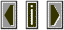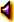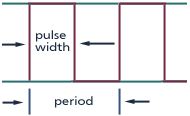Pulse waveA rectangular wave   form with an instant on and off slope and a variable on/off time or duty cycle.  Contains only odd harmonics.  If the length of the on time of the pulse is equal to the length of the off time (also known as a duty cycle of 1:1) then the pulse wave may also be called a square wave.pulse (square)The amplitude of a specific harmonic of a square wave (relative to the amplitude of the fundamental) is  the inverse of its harmonic number:  i.e  1/n where n is the partial number. Thus the fifth harmonic has 1/5th the amplitude of the fundamental.